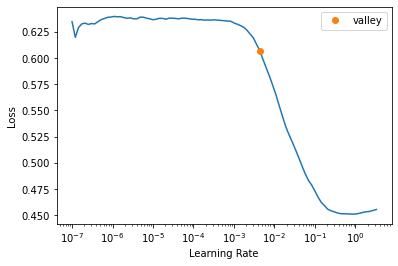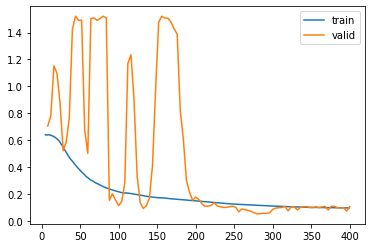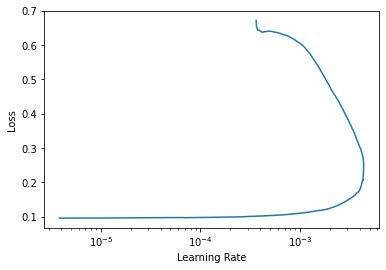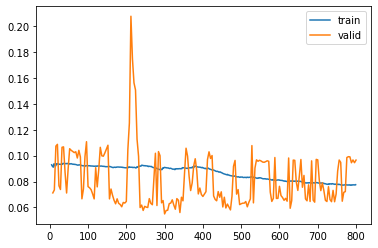# Object detection - Improve IoUs fit_one_cycle

Hello everyone,

I’m developing my first ever project on Deep Learning. I’m adapting the original notebook that was done by my professors.
The notebook is based on the detection of the the lungs on chest X-ray images.
I’ve 3 sets of images, the training set, the validation set and the test set.
I’ve managed to train a model successfully and to show the predicted bounding boxes of each lung on the validation set.
The model is a pre-trained model with it’s architecture being Resnet18.

I’ve done two trainings using the “fit_one_cycle” function. The first one with 100 epochs and with all the layers freezed except the last one. The second training is with 200 epochs and with all the layers unfreezed.
As a metric to both trainings I’ve set the IoU (Intersection over Union).
On the last epoch of each training, normally, I get around 0,75 of IoU. I say normally because the training/validation sets aren’t always the same on each execution, they can change because I’ve a RandomSplitter on the DataBlock object creation.

To try to improve the IoUs I’ve done multiple tests changing the learning rates, from having them hardcoded to setting a suggested learning rate like lr_valley or lr_min.
Now on each training I’ve set the parameter max_lr of fit_one_cycle to be “max_lr=lr_valley”.

My goal is to try to get higher IoUs, close to 0,90 or higher, so that the the predicted bounding boxes are closer to the targets.

I’ve 300 images annotated with a “.txt” for each image that contains the coordinates of the lungs.
The test set has 10 images. The rest of images are divided between the train set and the validation set. The train set has 261 images and the validation set has 29 images due to the RandomSplitter(0.1) of the DataBlock.

I don’t know how I can improve the IoUs.

I would appreciate if someone could give me suggestions on what I should change of my code to improve my model. I hope the concept of my project is understood, if there are some doubts I’ll give the appropiate details.

Thank you.

At the end of this message I post the key parts of my notebook and their respective outputs.

Bounding boxes:

``````# Generate the bounding boxes of an image with Path "f"
#   "f" is a Path and not a filename because the DataBlock object used next gets the paths
#   of all the images of the dataset & stores them in a list named "get_image_files"
#   For each image of the dataset, it's path is passed as parameter in get_bboxes to generate the target bounding boxes
def get_bboxes(f):

img = PILImage.create(path+f.name)

# Get the annotations of the bounding boxes of the lungs of the rx image with Path "f"
fullAnnot = np.genfromtxt(img2txt_name(f))

bboxes = np.zeros((2,4))

for i in range(len(fullAnnot)):
cx = int(fullAnnot[i]*img.size)
cy = int(fullAnnot[i]*img.size)

w = int(fullAnnot[i]*img.size)
h = int(fullAnnot[i]*img.size)

bbox = np.zeros(4)
bbox = float(cx-w/2.0) # minx
bbox = float(cy-h/2.0) # miny
bbox = float(cx+w/2.0) # maxX
bbox = float(cy+h/2.0) # maxY

bboxes[i] = bbox

return bboxes
``````

Data Block creation:

``````data = DataBlock(
blocks=(ImageBlock, CustomBboxBlock), # ImageBlock means type of inputs are images; BBoxBlock & BBoxLblBlock = type of targets are BBoxes & their labels
get_items=get_image_files,
n_inp=1, # number of inputs; it's 1 because the only inputs are the rx images (ImageBlock)
get_y= get_bboxes,
splitter = RandomSplitter (0.1), # split training/validation; parameter 0.1 means there will be 10% of validation images
batch_tfms= [*aug_transforms(do_flip=False, size=(120,160)), Normalize.from_stats(*imagenet_stats), BBoxReshape]
)
``````

IoU algorithm functions:

``````def intersection(preds, targs):
# preds and targs are of shape (validation dataset size, 8), pascal_voc format
max_xy_left = torch.min(preds[:, 2:4], targs[:, 2:4]) # get the min max_xy between preds & targs because it's the max_xy of the inner bbox
min_xy_left = torch.max(preds[:, :2], targs[:, :2]) # get the max min_xy between preds & targs because it's the min_xy of the inner bbox
inter_left = torch.clamp((max_xy_left - min_xy_left), min=0) # store the dimensions of the inner bbox as 2D Tensor (x,y)
# min=0 makes sure that none value of inter is < 0

max_xy_right = torch.min(preds[:, 6:], targs[:, 6:])
min_xy_right = torch.max(preds[:, 4:6], targs[:, 4:6])
inter_right = torch.clamp((max_xy_right - min_xy_right), min=0)

inter = (inter_left[:, 0] * inter_left[:, 1]) + (inter_right[:, 0] * inter_right[:, 1]) # get the total area of the inner bboxes (left & right)

return inter

def area(boxes):
area_left = ((boxes[:, 2]-boxes[:, 0]) * (boxes[:, 3]-boxes[:, 1])) # area of a rectangle: (maxX - minX) * (maxY - minY)
area_right = ((boxes[:, 6]-boxes[:, 4]) * (boxes[:, 7]-boxes[:, 5]))

return (area_left + area_right)

def union(preds, targs):
return area(preds) + area(targs) - intersection(preds, targs)

def IoU(preds, targs):
inter = intersection(preds, targs)
u = union(preds, targs)
return inter / u
``````

LungDetector model creation:

``````# Create the Lung Detector model from a pre-trained model

class LungDetector(nn.Module):
def __init__(self, arch=models.resnet18): # resnet18 has 18 lineal layers and it's the default arch if none arch is set as parameter
super().__init__()
self.cnn = create_body(arch) # cut off the body of a typically pretrained arch

# NOTE: What does forward function mean?
def forward(self, im): # NOTE: what does im mean?
x = self.cnn(im) # NOTE: why im is passed as parameter to cnn?
# NOTE: Understand what the following line does?, what is x.sigmoid_()?
return 2 * (x.sigmoid_() - 0.5) # (n_inp + bias) * n_out; bias = - 0.5, n_in = x.sigmoid_(), n_out = 2? (n_out I guess it should be 0 because now we don't have labels)
``````

Learner setup:

``````def loss_fn(preds, targs):
return L1Loss()(preds, targs.squeeze()) # compute Mean Absolute Error

learn = Learner(dls, LungDetector(arch=models.resnet50).cuda(), loss_func=loss_fn) # LungDetector.cuda() to work the model on GPU

learn.metrics = [lambda preds, targs: IoU(preds, targs.squeeze()).mean()]

``````

1st training (100 epochs):

``````learn.freeze_to(-1)
lr_valley = learn.lr_find()
````````````%time learn.fit_one_cycle(100, lr_max, div=12, pct_start=0.2)
``````

``````learn.recorder.plot_loss()
````````````learn.recorder.plot_lr_find()
``````2nd training (200 epochs):

``````learn.unfreeze()
lr_valley = learn.lr_find()
````````````max_lr = lr_valley
%time learn.fit_one_cycle(200, max_lr)
``````

``````learn.recorder.plot_loss()
``````Validation set predictions (PREDICTIONS ARE RED, TARGETS ARE GREEN):